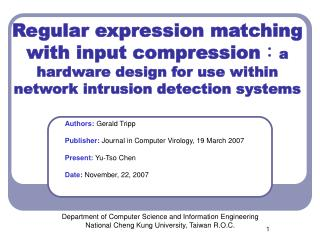DownloadDownload PresentationDepartment of Computer Science and Information Engineering

# Department of Computer Science and Information Engineering

Télécharger la présentation## Department of Computer Science and Information Engineering

- - - - - - - - - - - - - - - - - - - - - - - - - - - E N D - - - - - - - - - - - - - - - - - - - - - - - - - - -
##### Presentation Transcript

1. Regular expression matching with input compression：a hardware design for use within network intrusion detection systems Authors: Gerald Tripp Publisher: Journal in Computer Virology, 19 March 2007 Present:Yu-Tso Chen Date:November, 22, 2007 Department of Computer Science and Information Engineering National Cheng Kung University, Taiwan R.O.C.

2. Outline • 1. Introduction • 2. Definitions and problem description • 3. Matching of Individual Patterns • 4. Selective Grouping of Multiple Patterns • 5. Evaluation Result • 6. Conclusion

3. Introduction • Build a table based automata implement-ation but to use a form of input compression • The table based approach allow the system to be dynamically updated at run time • The input compression helps to make significant reductions in the automata memory requirements.

4. Outline • 1. Introduction • 2. Definitions and problem description • 3. Matching of Individual Patterns • 4. Selective Grouping of Multiple Patterns • 5. Evaluation Result • 6. Conclusion

5. Definitions and problem description • Simple table based implementations can require quite a lot of memory resources • REs themselves that often create automata with more node (and edges) • Tables required for implementing automata can have a high level of redundancy

6. Definitions and problem description (cont.) • For a Mealy machine, the amount of memory M in bits for a DFA with s states, i input bits and o output bits

7. Outline • 1. Introduction • 2. Definitions and problem description • 3. Regular expression implementation • 4. Input compression • 5. Evaluation Result

8. Packed array DFA implementation

9. Packed transition tables

10. Packed transition tables

11. Outline • 1. Introduction • 2. Definitions and problem description • 3. Regular expression implementation • 4. Input compression • 5. Evaluation Result

12. Input compression • Esn as the set of characters enabling the edge or edges between current state s and next state n • Complete set of edge sets Pa • Pa gives us the sets of characters that we are interested in for all DFA edges. • These sets may however have overlaps

13. Input compression • Pd is a set of disjoint sets of input characters

14. Example

15. Example (cont.)

16. Outline • 1. Introduction • 2. Definitions and problem description • 3. Regular expression implementation • 4. Input compression • 5. Evaluation Result

17. Evaluation Result